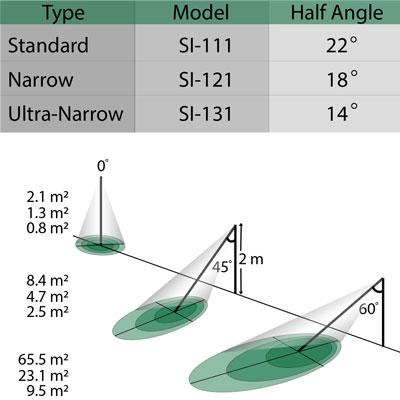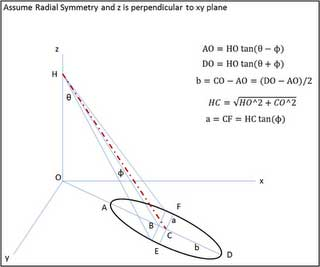# Field of View

FOV is reported as the half-angle of the apex of the cone formed by the target (cone base) and the infrared radiometer detector (cone apex). It ultimately determines how much surface area an IRR can see at any given distance and tilt angle. The target area is a circle from which 98 percent of the radiation viewed by the infrared radiometer detector is emitted. A center-weighted average of that target area is used to determine the mV signal. As the tilt angle is increased from zero degrees at a given height, the target area increases and transforms from being a circle to an ellipse as seen in the figure below.As the tilt angle is increased to high values such as 60 degrees, the target area increases significantly. In addition, the difference between target areas of the three models can become quite contrasting. Those attempting to measure an accurate average of the target area in question, whether it be a plant canopy or a highway, must determine if the desired target area covers the desired destination appropriately. Measuring more or less of the desired area can result in questionable results. To help with this uncertainty, Apogee has developed a simple tool that can be accessed on our website.This simple spreadsheet and illustration above allows the user to input the tilt angle (“Angle from Normal to Surface”), the height above surface, and the FOV depending on which IRR model is in use, as seen in the green cells. The result is the approximated target area as seen in the highlighted yellow cell. It should be noted that only the green cells should be edited, as not to interfere with cell calculation formulas. Due to the conical nature of the FOV, high tilt angles above approximately 65°, 70°, and 75° for the SI-111, SI-121, and SI-131 models, respectively, can yield impractical results in the spreadsheet due to “viewing” a near-infinite surface area. Likewise, Apogee recommends mounting the sensors below such angles. The FOV calculation spreadsheet can be used as a powerful tool in planning measurements such as deciding the model of sensor needed, an appropriate height and angle to mount your IRR, etc.